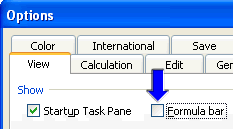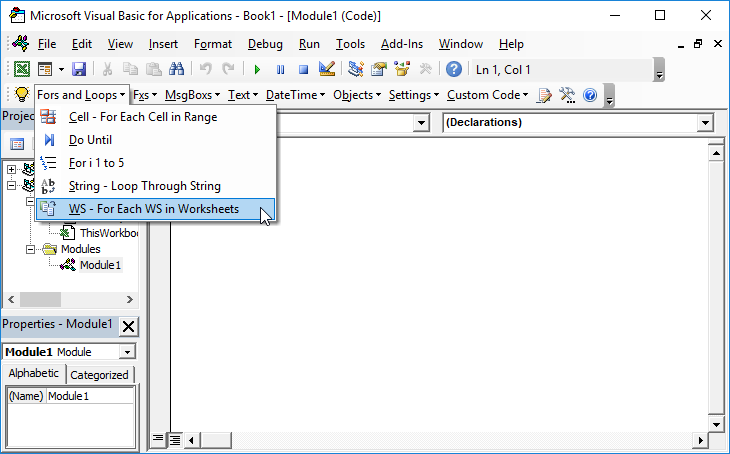# VBA – Turn Formula Bar Off (or On)

When creating an Excel template you may want to turn off the formula bar to limit unwanted user actions.

Alternatively, if you are using a spreadsheet and the formula bar is missing you may want to turn it back on.

## Turn Formula Bar On or Off From Excel

To toggle the formula bar On or Off:

On the Ribbon:
1. Click View
2. Check or Uncheck “Formula Bar” in the Show section
or you can use keyboard shortcuts: Alt > W > V > F## Turn Formula Bar On or Off with VBA

To do this from VBA:

### Macro to turn off the formula bar

``````Sub OffFormulaBar()
Application.DisplayFormulaBar = False
End Sub``````

### Macro to turn on the formula bar

``````Sub OnFormulaBar()
Application.DisplayFormulaBar = True
End Sub``````

## Expand or Collapse Formula Bar

You can also resize the Formula Bar by clicking and dragging at the bottom of the formula bar or by using this shortcut: CTRL + SHIFT + U (MAC: ^ + Shift + U).